# How to use Excel If Statement with Multiple Conditions Range [AND/OR]

To perform complicated and powerful data analysis, you need to test various conditions at a single point in time. The data analysis might require logical tests also within these multiple conditions.

For this, you need to perform Excel if statement with multiple conditions or ranges that include various If functions in a single formula.

Those who use Excel daily are well versed with Excel If statement as it is one of the most-used formula. Here you can check various Excel If or statement, Nested If, AND function, Excel IF statements, and how to use them. We have also provided a VIDEO TUTORIAL for different If Statements.

## Excel If Statement Multiple Conditions range

There are various If statements available in Excel. You have to know which of the Excel If you will work at what condition. Here you can check multiple conditions where you can use Excel If statement.

### 1) Excel If Statement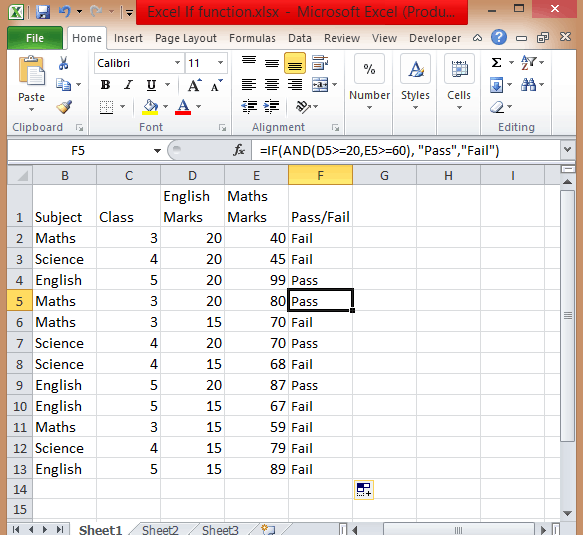If you want to test a condition to get two outcomes then you can use this Excel If statement.

`=If(Marks>=40, “Pass”)`

### 2) Nested If Statement

Let’s take an example that met the below-mentioned condition

• If the score is between 0 to 60, then Grade F
• If the score is between 61 to 70, then Grade D
• If the score is between 71 to 80, then Grade C
• If the score is between 81 to 90, then Grade B
• If the score is between 91 to 100, then Grade A

Then to test the condition the syntax of the formula becomes,

`=If(B5<60, “F”,If(B5<71, “D”, If(B5<81,”C”,If(B5<91,”B”,”A”)`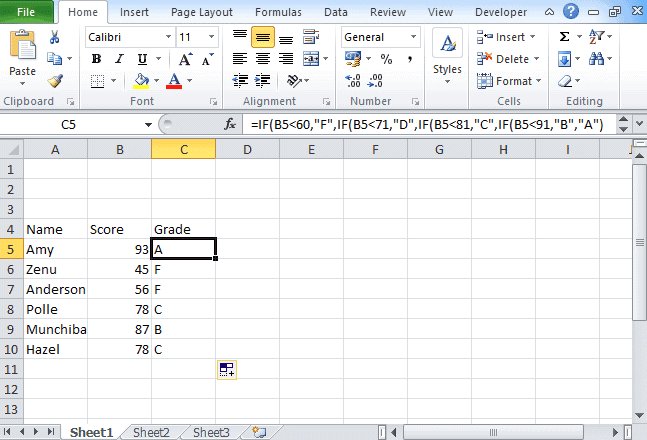### 3) Excel If with Logical Test

There are 2 different types of conditions AND and OR. You can use the IF statement in excel between two values in both these conditions to perform the logical test.

AND Function: If you are performing the logical test based on AND function, then excel will give you TRUE as an outcome in every condition else it will return false.

OR Function: If you are using OR condition for the logical test, then excel will give you an outcome as TRUE if any of the situations match else it returns false.

For this, multiple testing is to be done using AND and OR function, you should gain expertise in using either or both of these with IF statement. Here we have used if the function with 3 conditions.

#### How to apply IF & AND function in Excel

• To perform this multiple if and statements in excel, we will take the data set for the student’s marks that contain fields such as English and Math’s Marks.
• The score of the English subject is stored in the D column whereas the Maths score is stored in column E.
• Let say a student passes the class if his or her score in English is greater than or equal to 20 and he or she scores more than 60 in Maths.
• To create a report in matters of seconds, if formula combined with AND can suffice.
• Type =IF( Excel will display the logical hint just below the cell F2. The parameters of this function are logical_test, value_if_true, value_if_false.
• The first parameter contains the condition to be matched. You can use multiple If and AND conditions combined in this logical test.
• In the second parameter, type the value that you want Excel to display if the condition is true. Similarly, in the third parameter type the value that will be displayed if your condition is false.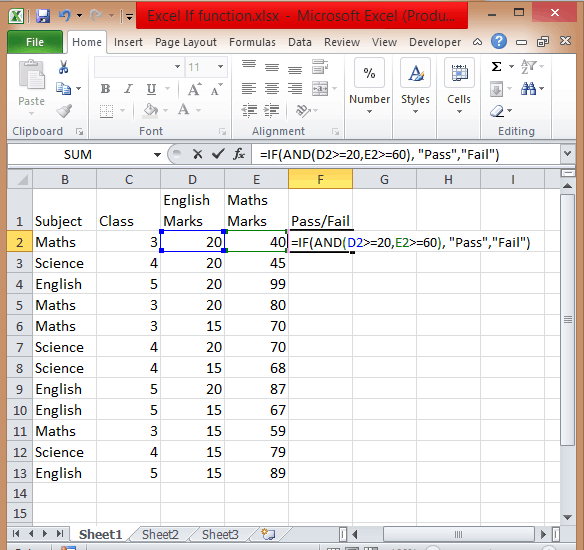• Apply If & And formula, you will get =IF(AND(D2>=20,E2>=60),”Pass”,”Fail”).• Add Pass/Fail column in the current table.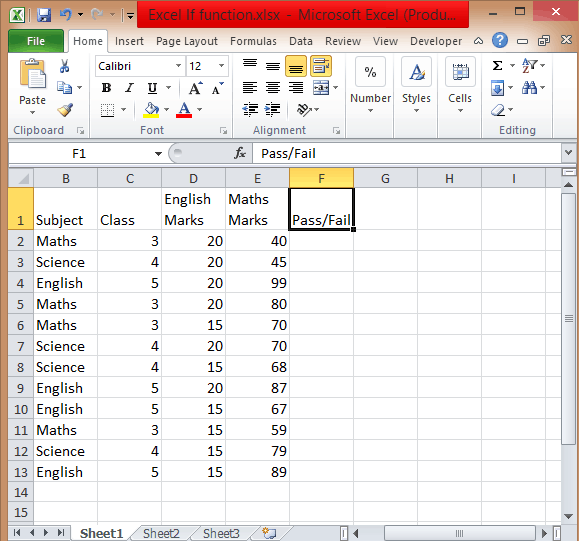• After you have applied this formula, you will find the result in the column.
• Copy the formula from cell F2 and paste in all other cells from F3 to F13.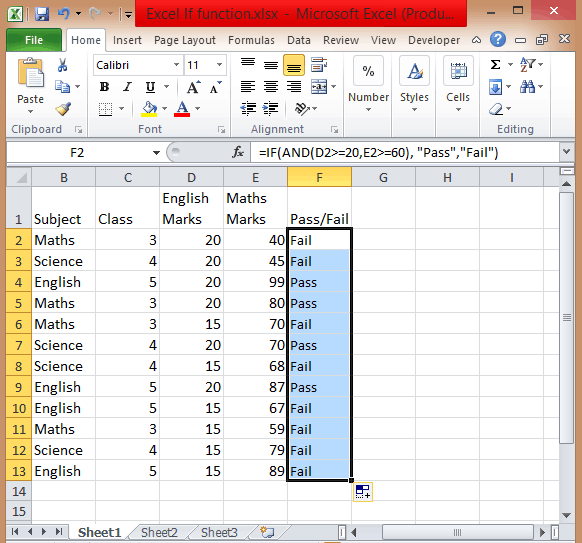#### How to use If with Or function in Excel

To use If and Or statement excel, you need to apply a similar formula as you have applied for If & And with the only difference is that if any of the condition is true then it will show you True.

To apply the formula, you have to follow the above process. The formula is =IF((OR(D2>=20, E2>=60)), “Pass”, “Fail”). If the score is equal or greater than 20 for column D or the second score is equal or greater than 60 then the person is the pass.

#### How to Use If with And & Or function

If you want to test data based on several multiple conditions then you have to apply both And & Or functions at a single point in time. For example,

Situation 1: If column D>=20 and column E>=60

Situation 2: If column D>=15 and column E>=60

If any of the situations met, then the candidate is passed, else failed. The formula is

`=IF(OR(AND(D2>=20, E2>=60), AND(D2>=20, E2>=60)), “Pass”, “Fail”).`

### 4) Excel If Statement with other functions

Above we have learned how to use excel if statement multiple conditions range with And/Or functions. Now we will be going to learn Excel If Statement with other excel functions.

• Excel If with Sum, Average, Min, and Max functions

Let’s take an example where we want to calculate the performance of any student with Poor, Satisfactory, and Good.

If the data set has a predefined structure that will not allow any of the modifications. Then you can add values with this If formula:

`=If((A2+B2)>=50, “Good”, If((A2+B2)=>30, “Satisfactory”, “Poor”))`

Using the Sum function,

`=If(Sum(A2:B2)>=120, “Good”, If(Sum(A2:B2)>=100, “Satisfactory”, “Poor”))`

Using the Average function,

`=If(Average(A2:B2)>=40, “Good”, If(Average(A2:B2)>=25, “Satisfactory”, “Poor”))`

Using Max/Min,

If you want to find out the highest scores, using the Max function. You can also find the lowest scores using the Min function.

`=If(C2=Max(\$C\$2:\$C\$10), “Best result”, “ “)`

You can also find the lowest scores using the Min function.

`=If(C2=Min(\$C\$2:\$C\$10), “Worst result”, “ “)`

If we combine both these formulas together, then we get

`=If(C2=Max(\$C\$2:\$C\$10), “Best result”, If(C2=Min(\$C\$2:\$C\$10), “Worst result”, “ “))`

You can also call it as nested if functions with other excel functions. To get a result, you can use these if functions with various different functions that are used in excel.

So there are four different ways and types of excel if statements, that you can use according to the situation or condition. Start using it today.

So this is all about Excel If statement multiple conditions ranges, you can also check how to add bullets in excel in our next post.

I hope you found this tutorial useful

You may also like the following Excel tutorials: